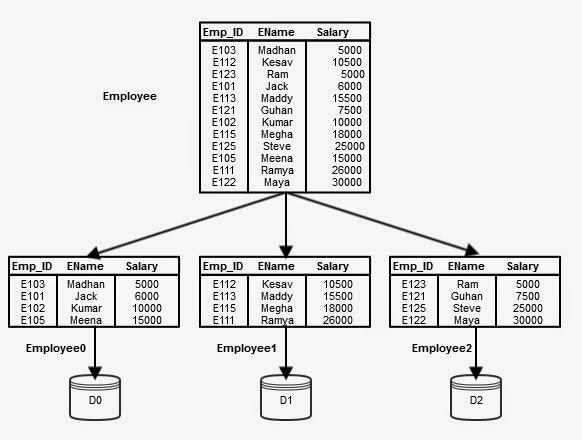Please visit, subscribe and share 10 Minutes Lectures in Computer Science

## How would we parallelize the SELECTION operation in an SQL query?

• Assume that the table is partitioned and stored in disks D0, D1, …, Dn-1 with processors P0, P1, …, Pn-1. For example, consider the following figure where the table Employee is partitioned using Round-robin partitioning technique.Figure 1 - Partitioned table Employee
• Recall the types of queries based on data access. Basically we have three types;
• Point queries – In point queries, the WHERE conditions are specified like “attribute_name = value” format.
• Range queries – In range queries, the WHERE conditions are specified like “attribute_name >= value AND attribute_name <= value”, or “attribute_name BETWEEN value1 and value 2”.
• Scanning the entire relation – In queries where the WHERE condition involves non-key attributes, then the system has to look for all the data in the specified table. This is called the relation scan.
With this information let us consider the following general syntax of any SQL select query;

SELECT list_of_attributes FROM table_name WHERE condition;

This query can be parallelized based on the condition given in the WHERE clause.

CASE 1: If the condition is of the form “a = value”, then;
If the given table is partitioned on a, then we need to execute the selection operation in only single processor where in the given attribute is partitioned.
CASE 2: If the condition is of the form “value1 <= a <= value2” (i.e, a falls in a range), then;
If the given relation is range partitioned on a, then we need to perform selection at all the processors wherever the range overlaps with the given range value1 to value2.
CASE 3: In all the other cases, the selection performed in parallel in all the processors. That is, for all the other type of queries, the selection can be done in parallel in all the processors, because, the data are partitioned over several processors.

As an example for CASE 3, let us assume a query which requests information from a table with a non-key attribute in the WHERE clause condition. Refer the Employee table in Figure 1.
SELECT * FROM emp WHERE ename = ‘Kumar’;
The query involves a condition on name attribute and we don’t create an index or key on name attribute as there are more such names. Hence, this query needs to examine all records of Employee table to give the final result. So, we can distribute the query to all the processors wherever Employee table's partitions are stored, and execute the query in parallel on all the processors. According to the example in Figure 1, Processors P0, P1, and P2 have to execute the query locally, even though the data available at D0 alone. The final result is generated from the local results of these processors. This way the parallelism for SELECTION operation can be achieved.
The same (CASE 3) is applicable for other type of actions like,

• If the table is partitioned based on an attribute other than the WHERE condition attribute,
• If the table is range partitioned on an attribute other than the range attribute specified in the query,
• If the table is partitioned on one attribute and the query involves one more attribute with and condition, etc.

#### Point to note:

• According to CASE 3, it means, we can achieve parallelism by exploiting all the processors.
• According to CASE 1, only one processor has to execute and it is known initially. That means, the other processors can effectively be used for executing other queries at that time.
• According to CASE 2, only few processors where the range of values stored need to execute. The other processors can be utilized for executing other queries.

## Featured Content

### Multiple choice questions in Natural Language Processing Home

MCQ in Natural Language Processing, Quiz questions with answers in NLP, Top interview questions in NLP with answers Multiple Choice Que...

data recovery